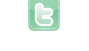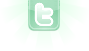This site requires JavaScript, please enable it in your browser!# pythagoras tree

public class Pythagoras2 extends Actor { /** * Act - do whatever the Pythagoras2 wants to do. This method is called whenever * the 'Act' or 'Run' button gets pressed in the environment. */ final int depth = 7; public Pythagoras2() { GreenfootImage gfim = new GreenfootImage(900,600); drawTree(gfim, 275, 500, 375, 500, 0); setImage(gfim); } private void drawTree(GreenfootImage gfim, float x1, float y1, float x2, float y2, int depth) { if (depth == 0) return; float dx = x2 - x1; float dy = y1 - y2; float x3 = x2 - dy; float y3 = y2 - dx; float x4 = x1 - dy; float y4 = y1 - dx; float x5 = x4 + 0.5F * (dx - dy); float y5 = y4 - 0.5F * (dx + dy); gfim.setColor(Color.BLUE); gfim.drawLine ((int)x1, (int)y1, (int)x2, (int)y2); gfim.drawLine ((int)x2, (int)y2, (int)x4, (int)y4); gfim.drawLine ((int)x4, (int)y4, (int)x3, (int)y3); gfim.drawLine ((int)x1, (int)y1, (int)x3, (int)y3); gfim.setColor(Color.GREEN); gfim.fillPolygon(new int {(int)x1,(int)x2,(int)x4,(int)x3}, new int {(int)y1,(int)y2,(int)y4,(int)y3}, 4); drawTree(gfim, x4, y4, x5, y5, depth + 1); drawTree(gfim, x5, y5, x3, y3, depth + 1); } } I started to make a Pythagorean tree from various source codes, it does not work but does not indicate any error, I think I'm on the right track, I hope I did not do anything Thank you for your help
Please use code tags for your code (see 'Posting code? read this!!" below the reply box. Then, try to explain what you thing each line (or set of lines) does in your code.
```import greenfoot.*;  // (World, Actor, GreenfootImage, Greenfoot and MouseInfo)

/**
* Write a description of class Pythagoras2 here.
*
* @author (your name)
* @version (a version number or a date)
*/
public class Pythagoras2 extends Actor
{
/**
* Act - do whatever the Pythagoras2 wants to do. This method is called whenever
* the 'Act' or 'Run' button gets pressed in the environment.
*/
final int depth = 7;

public Pythagoras2()
{
GreenfootImage gfim = new GreenfootImage(900,600);
drawTree(gfim, 275, 500, 375, 500, 0);
setImage(gfim);
}

private void drawTree(GreenfootImage gfim, float x1, float y1, float x2, float y2,
int depth)
{

if (depth == 0)
return;

float dx = x2 - x1;
float dy = y1 - y2;

float x3 = x2 - dy;
float y3 = y2 - dx;
float x4 = x1 - dy;
float y4 = y1 - dx;

float x5 = x4 + 0.5F * (dx - dy);
float y5 = y4 - 0.5F * (dx + dy);

gfim.setColor(Color.BLUE);
gfim.drawLine ((int)x1, (int)y1, (int)x2, (int)y2);
gfim.drawLine ((int)x2, (int)y2, (int)x4, (int)y4);
gfim.drawLine ((int)x4, (int)y4, (int)x3, (int)y3);
gfim.drawLine ((int)x1, (int)y1, (int)x3, (int)y3);

gfim.setColor(Color.GREEN);
gfim.fillPolygon(new int[] {(int)x1,(int)x2,(int)x4,(int)x3},
new int[] {(int)y1,(int)y2,(int)y4,(int)y3}, 4);

drawTree(gfim, x4, y4, x5, y5, depth + 1);
drawTree(gfim, x5, y5, x3, y3, depth + 1);

}

}
```
first of all line 9 = pythagoras2 is the superclass that inherits the actor class line 15 = int final variable that has an instance line 18 to 22 = constructor with class name gfim is the subject of greenfootimage drawTree with parameters x1, y1, x2, y2 setimage is a bit like setVisible line 25-26 = method with parameters line 29-30 = loop if equal 0 then return line 23 to 41 = variable with instance I guess dx means direction x line 45 sets the color line 46 to 49 = draw the lines x1, y1, etc which may form a square line 52 defines the color line 53-54 fills the polygon polygon with 4 parameters, I think I was wrong, I will have to put 3 parameters, which must form a triangle line 58-59 draws a tree with parameters that are shown in the class pythagoras2 if I'm not mistaken
You were not wrong -- a Pythagorean tree is made up of squares. You calculations are for a square that is oriented like a square (laying flat). They will not work on a square that is standing on a corner (one that looks more like a diamond). So, the second iteration will produce erroneous results which totally ruins any future iteration.
if I understand you correctly, it's not going to work because the pythagoras tree is made of squares and even with drawRect or drawPolygon to form a square it will not work.
ronald wrote...
if I understand you correctly, it's not going to work because the pythagoras tree is made of squares and even with drawRect or drawPolygon to form a square it will not work.
No. It will work if done correctly. Lines 40 and 41 have incorrect coordinates going into drawTree. on lines 58 and 59.
excuse me I misunderstood
I know what lines 40 and 41 are supposed to do; but, they aren't doing it. The 4 coordinates given the method via parameter arguments are for the endpoints of a line segment that makes the base of a new square. Lines 40 and 41 are supposed to compute the coordinates of a point that is one and one-half the length of the line segment "above" the midpoint of the segment. You need two points to get a midpoint; yet, those lines of code only utilize one point (y4). Also, you have dx and dy directly interacting with each other in those lines. That can not be good for anything except for maybe testing their relationship in an if conditional, but not in an assignment statement.
Thank you, I found the solution
You need to login to post a reply.